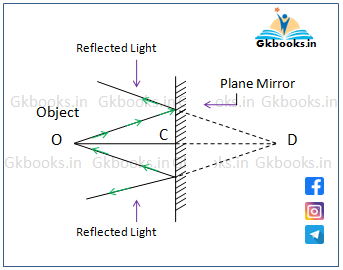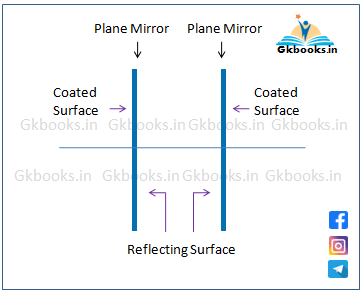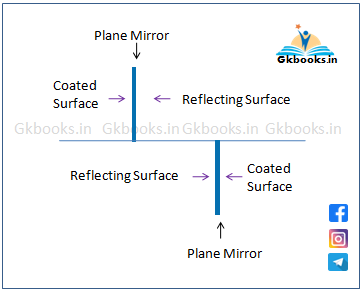# Image formed by Plane Mirror

Image formed by Plane Mirror‘ is an important topic under general science. For upcoming competitive exams like SSC, Banking, UPSC, Railways, State Level Exams general science is a vital subject. To cover all the topics of general science, we divided each chapter into several subtopics. The topic ‘Image formed by Plane Mirror ‘ comes under the chapter Ray optics of Physics.

## What is Mirror?

◘ A Mirror is a light reflecting, polished surface glass.
◘ A mirror reflects almost all light that is incident on it.
◘ The polished surface of the glass coated with reflective layer.

## Coating of Mirrors

◘ Applying a thin layer of metal (reflective layer) on one surface of a glass is known as a coating of the mirror.

◘ Metal plating techniques are used to give a thin coating to the metal.

◘ Metals used in Coating
• Silver
• Aluminum
• Gold
• Zinc
• Copper
• Platinum
• Tin

◘ Among the above list Silver and Aluminium are the most common and widely used metal in coating of mirrors.

## Silvering of Mirrors

◘ Silvering of Mirrors is a process in which a thin layer of silver applied on a surface of a glass, so that most of the incident light on it can be reflected.

◘ Glucose is used in the silvering of mirrors.

## Types of Mirror

◘ On the based on shape a mirror is classified into two types, namely ….
1. Plane mirror
2. Spherical mirror

### Plane Mirror

◘ When the reflecting surface of a mirror is flat (not curved) then it is called plane mirror.

♦ Properties of Plane mirror
◘ In case of a plane mirror the angle of reflection and the angle of incidence are equal.

◘ An image formed by a plane mirror is always virtual and erect.
◘ And the size of the image is equal in size to the object.

◘ The distance between the image from the mirror and the distance between the object from the mirror both are equal.

◘ Linear magnification produced by a plane mirror is 1.

◘ The minimum size of the mirror required to see the full image of an object is half of the height of the object.
◘ Focal length of plane mirrors in infinity. (that means Power is Zero)

### Imaged formed by Plane Mirror

◘ A plane mirror formed erect (Upright), virtual image.
◘ And the size of image is same as the object

◘ The image formed by a plane mirror is at the same distance inside the mirror as the object is in front of it.

• In the below image OC = CD
• ‘OC’ is the distance between the object and mirror and is CD is the distance between the image and the mirror.

◘ In order to view entire image of an object the minimum length of the plane mirror should be half of the length of the object.

• For an example, If your height is 5 feet and you want to see your full image in a plane mirror, then the length of the mirror should be 5/2 or 2.5 feet.Image formed by Plane mirror. (O is a point object , D image of the point object)

### Image formed by two Plane Mirror placed at a certain angle

◘ When two plane mirror is facing each other at an angle θ and object is placed between them, then

1. Number of images formed by two plane mirror is:
▲ (No. of Images) n = (m -1)  [Here, m = 3600 / θ]
★ If m is even
★ OR, the object lies symmetrically.

2. Number of images formed by the mirrors is:
▲ (No. of Images) n = m  [m = 3600 / θ]
★ If 3600 / θ is odd
★ OR, the object lies asymmetrically.

▲ Let’s Simplified the formula –
• At first calculate the value of m = (3600 / θ)

✪ If m is even, then (m -1) is the total number of images , irrespective of the position of the objects. (Symmetrical or Unsymmetrical)

✪ If m is odd then consider the following 2 cases..
◘ Case I
• If the object placed symmetrically then (m-1) is the actual number (n) of images.

◘ Case II
• If the object placed asymmetrically then n itself is the number (n=m) of images.

Numerical Example-
◘ Suppose two plane mirrors are perpendicular to each other or the angle between them is 900 .

◘ The number of images formed by the mirrors is __

◘ The Value of m = 360/90 = 4
◘ 4 is a even number, so the actual number of images is (4-1) =3

◘ Suppose the angle between two plane mirrors are 720

◘ The number of images formed by the mirrors is __

◘ Value of m = 360/72 = 5
◘ 5 is a odd number, so the actual number of images _

• n = (m – 1) = (5 – 1) = 4
• If the object placed symmetrically

• n = 5 (n=m)
• If the object placed asymmetrically

▲ Note –
◘ Two parallel plane mirror formed infinite numbers of images if the angle(θ) between them is 180 degrees and they are  facing each other like the below image.Number of images formed by two parallel mirror (When the  angle between them is 180 deg. (case 1)

◘ Two parallel plane mirror formed only 2 images if the angle (θ) between them is 180 degrees and they are  facing each other like the following image.The Number of Images formed by two parallel mirrors (Angle between them 180 degrees. case 2)

◘ Two parallel plane mirror formed only 2 images if they are perpendicular to each other or the angle (θ) between them is 90 degrees and they are facing each other like the following image.

### Spherical Mirror

◘ A spherical mirror is the small cut out portion, of a reflecting sphere.
◘ There are two types of spherical mirrors
1. Concave
2. Convex

♦ Concave mirror
◘ The spherical mirror whose inner surface is reflecting surface and outer surface is polished, is called concave mirror.

◘ Another name of concave mirror is Converging mirror , as because it is converge (light rays tend to meet at a point.) the beam of light after reflection.

♦ Convex mirror
◘ The spherical mirror whose outer surface is reflecting surface and the inner surface is polished, is called convex mirror.

◘ Another name of convex mirror is Diverging mirror because it generally diverges ( separate the rays of light from another) the beam of light after reflection.

## Usage of Mirror

♦ Plane mirror
◘ Some of the common uses of Plane mirrors are _
• As a looking glass.
• Used in torch lights.
• Used in solar cookers.
• Used in constructing periscope. (2 plane mirror is used)
• Used in making kaleidoscope toys.

♠ The Kaleidoscope is a an optical instrument which produces beautiful color patterns.
♠ It has two or more reflecting surface which is placed such an angle so that it can produce multiple symmetrical patterns.

♦ Concave mirror
◘ Some of the common uses of concave mirrors are _
• Searchlights
• Shaving mirrors
• makeup mirrors
• Satellite dishes
• dental mirrors

♦ Convex mirror
◘ Convex mirrors are commonly used in –
• Rear view mirror in vehicles.
• Used as a Security mirror in shop
• Used in interior design

◘ Convex mirror provides a much wider angle of view compared to plane mirror.